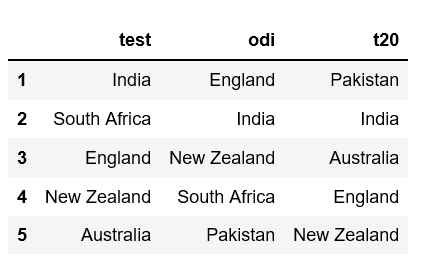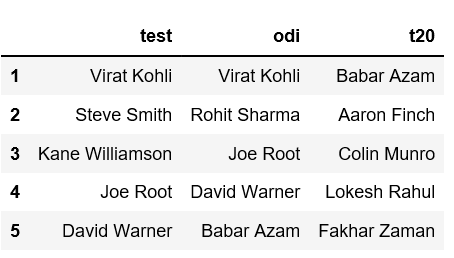# Python | Create a Pandas Dataframe from a dict of equal length lists

Given a dictionary of equal length lists, task is to create a Pandas DataFrame from it.

There are various ways of creating a DataFrame in Pandas. One way is to convert a dictionary containing lists of equal lengths as values. Let’s discuss how to create a Pandas Dataframe from a dict of equal length lists with help of examples.

Example #1: Given a dictionary which contains format of cricket as keys and list of top five teams as values.

 `# Import pandas package  ` `import` `pandas as pd ` ` `  `# Define a dictionary containing ICC rankings ` `rankings ``=` `{``'test'``: [``'India'``, ``'South Africa'``, ``'England'``, ` `                            ``'New Zealand'``, ``'Australia'``], ` `              ``'odi'``: [``'England'``, ``'India'``, ``'New Zealand'``, ` `                            ``'South Africa'``, ``'Pakistan'``], ` `               ``'t20'``: [``'Pakistan'``, ``'India'``, ``'Australia'``,  ` `                              ``'England'``, ``'New Zealand'``]} ` ` `  `# Convert the dictionary into DataFrame ` `rankings_pd ``=` `pd.DataFrame(rankings) ` ` `  `# Increment the index so that index  ` `# starts at 1 (starts at 0 by default)  ` `rankings_pd.index ``+``=` `1` ` `  `rankings_pd `

Output:Example #2: Given three lists `test_batsmen`, `odi_batsmen`, `t20_batsmen`. So we first need to convert this data into a dictionary and then convert the dictionary into DataFrame.

 `# Import pandas package  ` `import` `pandas as pd ` ` `  `# Lists of top 5 batsmen for each format ` `test_batsmen ``=` `[``'Virat Kohli'``, ``'Steve Smith'``, ``'Kane Williamson'``, ` `                                     ``'Joe Root'``, ``'David Warner'``] ` `odi_batsmen ``=` `[``'Virat Kohli'``, ``'Rohit Sharma'``, ``'Joe Root'``, ` `                            ``'David Warner'``, ``'Babar Azam'``] ` `t20_batsmen ``=` `[``'Babar Azam'``, ``'Aaron Finch'``, ``'Colin Munro'``, ` `                           ``'Lokesh Rahul'``, ``'Fakhar Zaman'``] ` ` `  `# Define a dictionary containing ICC rankings for batsmen ` `rankings_batsmen ``=` `{``'test'``: test_batsmen, ` `                      ``'odi'``: odi_batsmen, ` `                      ``'t20'``: t20_batsmen} ` ` `  `# Convert the dictionary into DataFrame ` `rankings_batsmen_pd ``=` `pd.DataFrame(rankings_batsmen) ` ` `  `# Increment the index so that index ` `# starts at 1 (starts at 0 by default)  ` `rankings_batsmen_pd.index ``+``=` `1` ` `  `rankings_batsmen_pd `

Output:My Personal Notes arrow_drop_upCheck out this Author's contributed articles.

If you like GeeksforGeeks and would like to contribute, you can also write an article using contribute.geeksforgeeks.org or mail your article to contribute@geeksforgeeks.org. See your article appearing on the GeeksforGeeks main page and help other Geeks.

Please Improve this article if you find anything incorrect by clicking on the "Improve Article" button below.

Article Tags :

6

Please write to us at contribute@geeksforgeeks.org to report any issue with the above content.